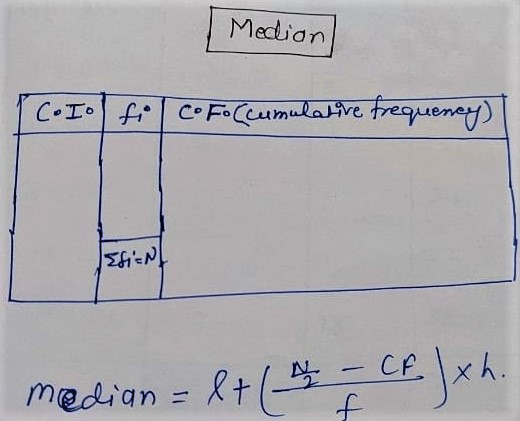# What is Median in Statistics with Example

In the measurement of central tendency, the Median has an important role, Median divides Numerical data into two parts from the middle that’s why it’s called the median, But during finding the median it is important for which type of data you have to find :

For Ungrouped or discrete data, this is important that first of all, you will arrange the data in ascending order or descending  and then find the median here are the two cases as follows:

case1:

If  n = number of terms/observations (even)   then,

Median = [ (n/2)th  term + { (n/2)th +1}term]/2

case2:

If  n = number of terms /observation(odd)

Median = (n/2)th  term

By the following example above points will be clear

Example1:

The following Points scored by a Kho-Kho team in a series of marches are as follows:

16    1    6    26    14    7    10    9    15    8    24    47    10    6    9    28    23    11    20     18

Find the median of the points scored by the team.

Solution:

first of all, arrange the following points scored by the team in ascending order

1    6    6    7    8    9    9   10    10   11    14    15    16    18    20   23   24   26   28   47

n = total number of terms

here n = 20 (which is even)

if the number of terms is even in this case median is the mean of (n/2)th  term and (n+1/2)th term

Median = [ (n/2)th  term + { (n/2)th + 1}term]/2

=> Median = [(20/2)th  term + {(20/2)th +1}term]/2

=> Median = [(10)th  term + {(10)th + 1}term]/2

=> Median = [(10)th  term + {11th}term]/2

=> Median = [11 + 14]/2

=> Median = /2

=> Median = 17.5

Example 2:

The following number of goals were scored by a team in a series of 11 matches:

2,  3,  1,  5,  0,  2,  6,  3,  2,  6,  4

Solution:

Arrange in ascending order

0,    1,    2,    2,    2,    3,    3,   4,    5,   6,    6

n = total number of goals(terms)

n = 11(odd)

Median = (n+1)th /2 term

Median = [(11+1)/2]th  term

Median = (12/2)th  term

Median = (6)th  term

Median = 3

Example 3:

The marks obtained by 30 students of class 9th of a certain school in a Mathematics paper consisting of 100 marks are presented in the table below. find the mean of the marks obtained by the students.

 Marks obtained 20 29 28 33 42 38 43 25 Number of students 6 28 24 15 2 4 1 20

First of all, we arrange  the marks in ascending order and rearrange a frequency table as follows

 Marks obtained Number Of Students C.F.(cumulative Frequency) 20 25 28 29 33 38 42 43 6 20 24 28 15 4 2 1 6 6+20=26 26+24=50 (50th observation) 50+28=78 (51st observation) 78+15=93 93+4=97 97+2=99 99+1=100 Total=100

Here n = 100 (even)

Median = [ (n/2)th  term + { (n/2)th  + 1}term]/2

(100/2)th observation = 50th observation = 28

{ (100/2)th + 1}observation]/2 = {50 + 1}th observation = 51th observation = 29

Median = [ (n/2)th  term + { (n/2)th + 1}term]/2

Median = [50th observation + 51th observation]/2

If the Data are on a huge scale and the difference between the least observation and the highest observation is also large in this situation we will make a grouped frequency distribution table to find the median.

For Example:

The maths guides are sold in a shop for a month(30 days) and are as follows:

6, 12, 5, 7, 17, 3, 25  2, 16, 20, 3, 9,  4, 12, 7, 8, 5, 8, 3, 4, 12, 13, 14, 17, 7, 0, 7, 17, 9, 10

The following table is continuously grouped frequency distribution table

 Class-interval frequency 0-5 5-10 10-15 15-20 20-25 25-30 7 11 6 4 1 1

we can find the median for grouped frequency distribution table by the following method:Class-interval frequency(fi) C.F.(cumulative frequency) 0-5 5-10 ……… 4 5 ……. ∑fi=n 4 4+5=9 ………

Median Class interval = ∑fi/2 = n/2 watch in cf whose cumulative frequency is greater than and nearest to n/2

Median = l+[(n/2-cf)/f]×h

Here

l = Lower limit of the median class interval,

n = number of observation

cf = cumulative frequency of class preceding the median class-interval

h = class size (assuming class size to be equal)

f = Mode class interval frequency

For Example:

The following table gives the distribution of the time of 400 neon lamps:

 lifetime (in hours) Number of lamps 1500-2000 2000-2500 25000-3000 3000-3500 3500-4000 4000-4500 4500-5000 14 56 60 86 74 62 48

Find the median lifetime of a lamp.

Solution:

 lifetime(in hours) Number of lamps (frequency=fi) C.F.(cumulative frequency) 1500-2000 2000-2500 25000-3000 3000-3500 3500-4000 4000-4500 4500-5000 14 56 60 86 74 62 48 14 14+56=70 70+60=130 130+86=216 (median C.I.) 216+74=290 290+62=352 352+48=400 ∑fi=400

for the median class interval (C.I.)

∑fi/2 = n/2 = 400/2 = 200 (watch in c.f. whose cumulative frequency is greater than and nearest to n/2)

Median class interval =  3000-3500        86            216

Now

l = Lower limit of the median class interval=3000,

n = number of observation=400

cf = cumulative frequency of class preceding the median class-interval-=130

h = class size (assuming class size to be equal)=500

f = Mode class interval frequency=86

Median = l + [(n/2 – cf)/f] × h

Median= 3000 + [(400/2  –  130)/86] × 500

=> median = 3000 + [ (200 – 130)/86 ] × 500

=> median = 300 + [70/86] × 500

=> median = 3000 + [0.813] × 500

=> median = 3000 + 406.97

=> median = 3406.97